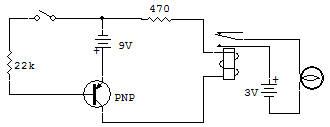Recherche personnalisé / personalised search

Electronic Relay SwitchingThis project is a demonstration of electronic relay control. We will consider how to energize a Relay by use of a switching Transistor, and why this type of circuit is used.

Let's first consider the current levels in this demonstration circuit. To start with, the 3V Lamp draws over 300mA (milliamps) from the 3V Battery, the Relay circuit draws about 40mA from the 9V Battery, and the Key circuit draws only about 1mA.

Notice that a circuit of relatively high current (300mA) can be controlled by a switch that only has to pass a very small current (1mA). This is the basic reason why electronic relay control is used. Of course, in industrial power machinery the current levels are in amps (amps = 1000 X mA).

The relays used around your house for this purpose include those in the washing machine, dryer, dishwasher, electric range, furnace, automobile, etc. So you see this is a popular method of switching. It allows small switches and controls to control large amounts of power.

The Relay has many turns of fine wire in the field coil so that only 30mA or so is required to energize it and thus activate the contacts. Current greater than this is always applied to obtain reliable operation. For example, when used with 9V the current is about 40mA.

The Transistor can operate so that it acts like a switch between the C (collector) and E (emitter) leads. When no current is applied to the base (B), the Transistor is OFF. When sufficient current is supplied to the base, the Transistor is ON. The amount of current required in the base circuit is dependent on the current gain (called Beta) of the Transistor.

Different Transistors have different gains or current ratios. The 2SA has (typically) a Beta of 200 or so. This means that the base current must be at least 1/200 of the collector current. To insure that circuit will consistently turn ON, it is best to assume a Beta (gain of Transistor) of no more than 40. The required base current then is 40mA divided by 40 = 1mA. The 10K Resistor in series with the 9V Battery is able to supply about 1mA of current, so operation is assured. If you have a VOM you can check these currents directly in this circuit.

Recherche personnalisée